# Intermediate Algebra Tutorial 27

Intermediate Algebra
Tutorial 27: The GCF and Factoring by Grouping

WTAMU > Virtual Math Lab > Intermediate AlgebraLearning Objectives

After completing this tutorial, you should be able to:
1. Find the Greatest Common Factor (GCF) of a polynomial.
2. Factor out the GCF of a polynomial.
3. Factor a polynomial with four terms by grouping.Introduction

Factoring is to write an expression as a product of factors.  For example, we can write 10 as (5)(2), where 5 and 2 are called factors of 10.  We can also do this with polynomial expressions.  In this tutorial we are going to look at two ways to factor polynomial expressions, factoring out the greatest common factor and factoring by grouping.  In the next two tutorials we will add on other types of factoring.  Something to look forward to!  By the time I'm are through with you, you will be a factoring machine.  Others will be asking you for help with factoring.

Basically, when we factor, we reverse the process of multiplying the polynomial which was covered  in Tutorial 26: Multiplying Polynomials.Tutorial

Greatest Common Factor (GCF)

The GCF for a polynomial is the largest monomial that divides (is a factor of) each term of the polynomial.Example 1:   Find the GCF of the list of monomials: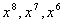We need to figure out what the largest monomial that we can divide out of each of these terms would be.  Note how they all have an x, so it look like x will be involved.

The exponents on the x’s are 8, 7, and 6.  We have to decide which exponent we are going to use.  If we use the exponent 8, we are in trouble.  We cannot  divide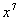or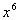by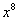, we don’t have enough x’s to do that.  But, if we use, we would have a monomial that we could divide out of ALL the terms.

Hence our GCF is.

Note that if all terms have the same variable, the GCF for the variable part is that variable raised to the lowest exponent that is listed.Example 2:   Find the GCF of the list of monomials: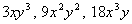We need to figure out what the largest monomial that we can divide out of each of these terms would be.  What do you think?

Let’s first look at the numerical part.  We have a 3, 9, and 18.  The largest number that can be divided out of those numbers is 3.

So our numerical GCF is 3.

Now onto the variable part.  It looks like each term has an x and a y.  In both cases the lowest exponent is 1.

So the GCF of our variable part is xy.

Putting this together we have a GCF of 3xy.

Factoring out the GCF

Step 1:  Identify the GCF of the polynomial.

Step 2:   Divide the GCF out of every term of the polynomial.

This process is basically the reverse of the distributive property found in Tutorial 5: Properties of Real Numbers.Example 3:   Factor out the GCF: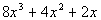Step 1:  Identify the GCF of the polynomial.

The largest monomial that we can factor out of each term is 2x.

Step 2:  Divide the GCF out of every term of the polynomial.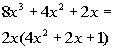*Divide 2x out of every term of the poly.

Be careful.  If a term of the polynomial is exactly the same as the GCF, when you divide it by the GCF you are left with 1, NOT 0.  Don’t think, 'oh I have nothing left', there is actually a 1.  As shown above when we divide 2x by 2x we get 1, so we need a 1 as the third term inside of the (  ).

Note that if we multiply our answer out, we should get the original polynomial.  In this case, it does check out.  Factoring gives you another way to write the expression so it will be equivalent to the original problem.Example 4:   Factor out the GCF: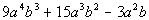Step 1:  Identify the GCF of the polynomial.

The largest monomial that we can factor out of each term is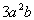.

Step 2:  Divide the GCF out of every term of the polynomial .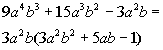*Divideout of every term of the poly.

Note that if we multiply our answer out, we do get the original polynomial.Example 5:   Factor out the GCF: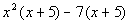This problem looks a little different, because now our GCF is a binomial. That is ok, we treat it in the same manner that we do when we have a monomial GCF.

Note that this is not in factored form because of the minus sign we have before the 7 in the problem.  To be in factored form, it must be written as a product of factors.

Step 1:  Identify the GCF of the polynomial.

This time it isn't a monomial but a binomial that we have in common.

Our GCF is (x + 5).

Step 2:  Divide the GCF out of every term of the polynomial .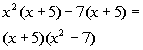*Divide (x + 5) out of both parts

When we divide out the (x + 5) out of the first term, we are left with x squared.  When we divide it out of the second term, we are left with -7.

That is how we get the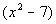for our second (  ).

Factoring a Polynomial with
Four Terms by Grouping

In some cases there is not a GCF for ALL the terms in a polynomial.  If you have four terms with no GCF, then try factoring by grouping.

Step 1: Group the first two terms together and then the last two terms together.

Step 2: Factor out a GCF from each separate binomial.

Step 3: Factor out the common binomial.Example 6:   Factor by grouping: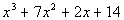Note how there is not a GCF for ALL the terms.  So let’s go ahead and factor this by grouping.

Step 1: Group the first two terms together and then the last two terms together.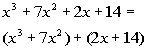*Two groups of two terms

Step 2: Factor out a GCF from each separate binomial.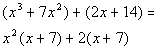*Factor out an x squared from the 1st (  )
*Factor out a 2 from the 2nd (  )

Step 3: Factor out the common binomial.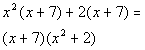*Divide (x + 7) out of both parts

Note that if we multiply our answer out, we do get the original polynomial.Example 7:   Factor by grouping: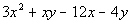Note how there is not a GCF for ALL the terms.  So let’s go ahead and factor this by grouping.

Step 1: Group the first two terms together and then the last two terms together.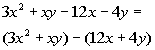*Two groups of two terms

Be careful.  When the first term of the second group of two has a minus sign in front of it, you want to put the minus in front of the second (   ).  When you do this you need to change the sign of both terms of the second (  ) as shown above.

Step 2: Factor out a GCF from each separate binomial.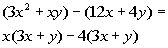*Factor out an x from the 1st (  )
*Factor out a 4 from the 2nd (  )

Step 3: Factor out the common binomial.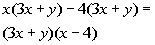*Divide (3x + y) out of both parts

Note that if we multiply our answer out that we do get the original polynomial.Practice Problems

These are practice problems to help bring you to the next level.  It will allow you to check and see if you have an understanding of these types of problems. Math works just like anything else, if you want to get good at it, then you need to practice it.  Even the best athletes and musicians had help along the way and lots of practice, practice, practice, to get good at their sport or instrument.  In fact there is no such thing as too much practice.

To get the most out of these, you should work the problem out on your own and then check your answer by clicking on the link for the answer/discussion for that  problem.  At the link you will find the answer as well as any steps that went into finding that answer.Practice Problems 1a - 1d: Factor.

1a.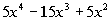1b.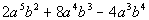1c.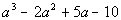1d.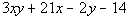Need Extra Help on these Topics?

The following are webpages that can assist you in the topics that were covered on this page:

http://www.purplemath.com/modules/simpfact.htm
This webpage helps you with factoring out the GCF.

http://www.mathpower.com/tut111.htm

Go to Get Help Outside the Classroom found in Tutorial 1: How to Succeed in a Math Class for some more suggestions.Last revised on July 15, 2011 by Kim Seward.